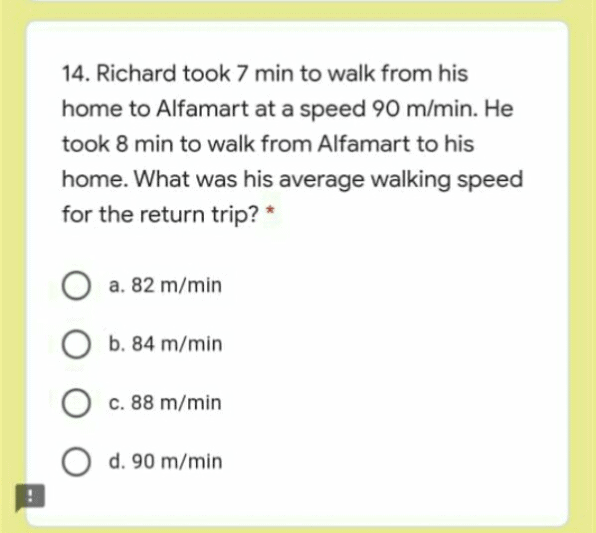# QuestionHai…can someone help me. Is the answer 84 m/min?

Distance

= Speed x Time

= 90m/min x 7min

=630m

Speed (For Alfamart back to home)

= Distance / Time

= 630m / 8min

= 78.75m/min

However if it is for the entire trip,

Average Speed

= Total Distance / Total Time

= 1260m / 15min

= 84m/min